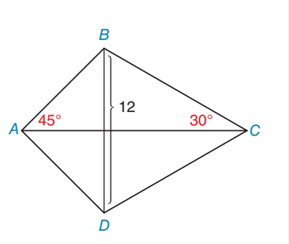Chapter 8.2, Problem 17EElementary Geometry For College St...

7th Edition
Alexander + 2 others
ISBN: 9781337614085

Solutions

Chapter
SectionElementary Geometry For College St...

7th Edition
Alexander + 2 others
ISBN: 9781337614085
Textbook Problem

In Exercises 13 to 18, find the area of the given polygon.kite A B C D with B D = 12 m ∠ B A C = 45 ∘ ,   m ∠ B C A = 30 ∘

To determine

To Find:

Area of the kite.

Explanation

Formula Used:

The area A of a kite whose diagonals have lengths d1 and d2 is given by A=12d1d2.

Calculation:

It is given that the kite ABCD has one of the diagonals BD=d1=12 and mBAC=45°,mBCA=30°.

Let’s draw another diagonal AC so that the diagonals BD and AC meet at a point be 'O'.

AC=AO+OC  (1)

In kite, the diagonals d1 and d2 are perpendicular bisector of each other. That is BOA=BOC=90°.

As BD is the perpendicular bisector BO, BO=BD2=122=6

Now consider the triangle AOB,

ABO=180-(BOA+BAO)

ABO=180-(90°+45°)

ABO=45°

Therefore, the triangle AOB is a 45°:45°:90° right triangle

Still sussing out bartleby?

Check out a sample textbook solution.

See a sample solution

The Solution to Your Study Problems

Bartleby provides explanations to thousands of textbook problems written by our experts, many with advanced degrees!

Get Started

Find more solutions based on key concepts